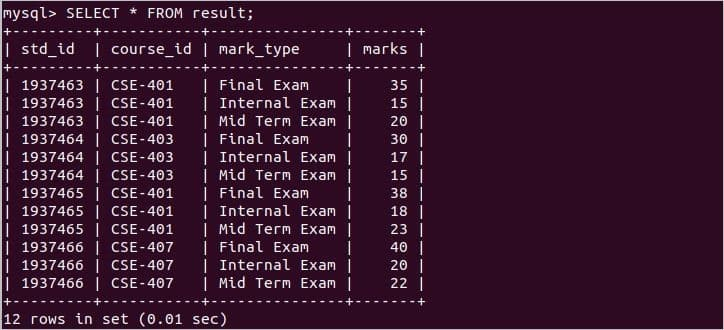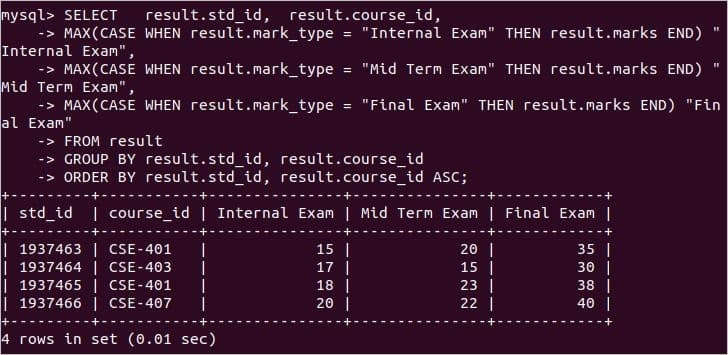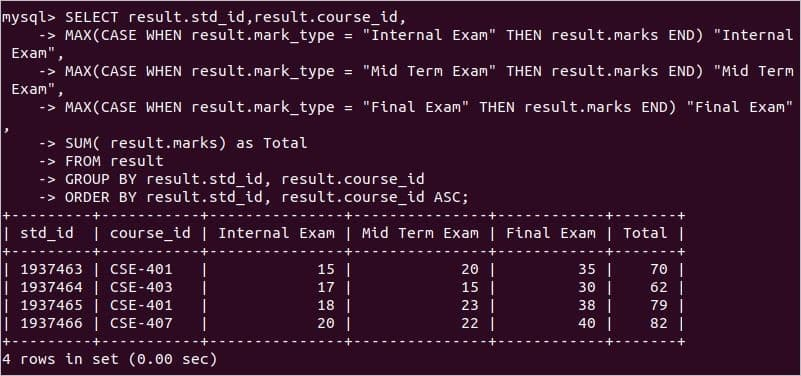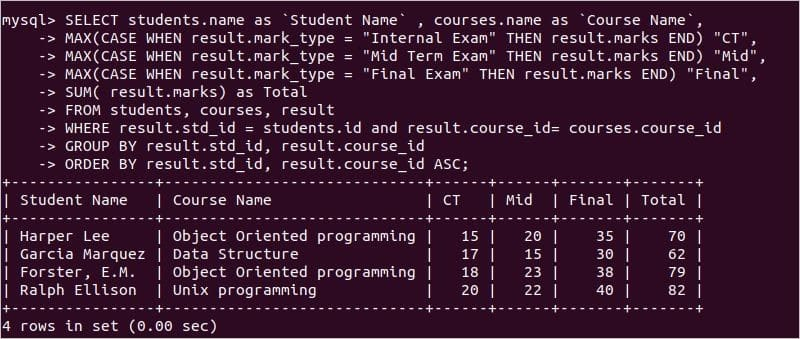# MySQL Pivot: rotating rows to columns

A database table can store different types of data and sometimes we need to transform row-level data into column-level data. This problem can be solved by using the PIVOT() function. This function is used to rotate rows of a table into column values. But this function is supported by very few database servers, such as Oracle or SQL Server. If you want to do the same task in the MySQL database table then you have to write the SELECT query using the CASE statement to rotate the rows into columns. The article shows the way to do the task of PIVOT() function within related MySQL database tables.

### Prerequisite:

You have to create a database and some related tables where rows of one table will be converted into the columns like PIVOT() function. Run the following SQL statements to create a database named ‘unidb’ and create three tables named ‘students’, ‘courses’ and ‘result’. students and result tables will be related by one-to-many relationship and courses and results tables will be related by one-to-many relationship here. CREATE statement of the result table contains two foreign key constraints for the fields, std_id, and course_id.

CREATE DATABASE unidb;
USE unidb;

CREATE TABLE students (
id INT PRIMARY KEY,
name varchar(50) NOT NULL,
department VARCHAR(15) NOT NULL);

CREATE TABLE courses (
course_id VARCHAR(20) PRIMARY KEY,
name varchar(50) NOT NULL,
credit SMALLINT NOT NULL);

CREATE TABLE result(
std_id INT NOT NULL,
course_id VARCHAR(20) NOT NULL,
mark_type VARCHAR(20) NOT NULL,
marks SMALLINT NOT NULL,
FOREIGN KEY (std_id) REFERENCES students(id),
FOREIGN KEY (course_id) REFERENCES courses(course_id),
PRIMARY KEY (std_id, course_id, mark_type));

Insert some records into students, courses and result tables. The values must be inserted into the tables based on the restrictions set at the time of table creation.

INSERT INTO students VALUES
( '1937463', 'Harper Lee', 'CSE'),
( '1937464', 'Garcia Marquez', 'CSE'),
( '1937465', 'Forster, E.M.', 'CSE'),
( '1937466', 'Ralph Ellison', 'CSE');

INSERT INTO courses VALUES
( 'CSE-401', 'Object Oriented programming', 3),
( 'CSE-403', 'Data Structure', 2),
( 'CSE-407', 'Unix programming', 2);

INSERT INTO result VALUES
( '1937463', 'CSE-401','Internal Exam' ,15),
( '1937463', 'CSE-401','Mid Term Exam' ,20),
( '1937463', 'CSE-401','Final Exam', 35),
( '1937464', 'CSE-403','Internal Exam' ,17),
( '1937464', 'CSE-403','Mid Term Exam' ,15),
( '1937464', 'CSE-403','Final Exam', 30),
( '1937465', 'CSE-401','Internal Exam' ,18),
( '1937465', 'CSE-401','Mid Term Exam' ,23),
( '1937465', 'CSE-401','Final Exam', 38),
( '1937466', 'CSE-407','Internal Exam' ,20),
( '1937466', 'CSE-407','Mid Term Exam' ,22),
( '1937466', 'CSE-407','Final Exam', 40);

Here, result table contains multiple same values for std_id, mark_type and course_id columns in each row. How to convert these rows into columns of this table for displaying the data in a more organized format is shown in the next part of this tutorial.

### Rotate rows to columns using CASE statement:

Run the following simple SELECT statement to display all records of the result table.

SELECT * FROM result;

The output shows the four student’s marks for three exam types of three courses. So the values of std_id, course_id and mark_type are repeated multiple times for the different students, courses and exam types.The output will be more readable if the SELECT query can be written more efficiently by using the CASE statement. The following SELECT with the CASE statement will transform the repeating values of the rows into the column names and display the content of the tables in a more understandable format for the user.

SELECT result.std_id, result.course_id,
MAX(CASE WHEN result.mark_type = "Internal Exam" THEN result.marks END) "Internal Exam",
MAX(CASE WHEN result.mark_type = "Mid Term Exam" THEN result.marks END) "Mid Term Exam",
MAX(CASE WHEN result.mark_type = "Final Exam" THEN result.marks END) "Final Exam"
FROM result
GROUP BY result.std_id, result.course_id
ORDER BY result.std_id, result.course_id ASC;

The following output will appear after running the above statement which is more readable than the previous output.### Rotate rows to columns using CASE and SUM():

If you want to count the total number of each course of every student from the table then you have to use the aggregate function SUM() group by std_id and course_id with the CASE statement. The following query is created by modifying the previous query with SUM() function and GROUP BY clause.

SELECT result.std_id,result.course_id,
MAX(CASE WHEN result.mark_type = "Internal Exam" THEN result.marks END) "Internal Exam",
MAX(CASE WHEN result.mark_type = "Mid Term Exam" THEN result.marks END) "Mid Term Exam",
MAX(CASE WHEN result.mark_type = "Final Exam" THEN result.marks END) "Final Exam",
SUM( result.marks) as Total
FROM result
GROUP BY result.std_id, result.course_id
ORDER BY result.std_id, result.course_id ASC;

The output shows a new column named Total that is displaying the sum of the marks of all exam types of each course obtained by each particular student.### Rotate rows to columns in multiple tables:

The previous two queries are applied to the result table. This table is related to the other two tables. These are students and courses. If you want to display the student name instead of student id and course name instead of course id then you have to write the SELECT query using three related tables, students, courses and result. The following SELECT query is created by adding three table names after FORM clause and setting appropriate conditions in the WHERE clause to retrieve the data from the three tables and generate more appropriate output than the previous SELECT queries.

SELECT students.name as `Student Name` , courses.name as `Course Name`,
MAX(CASE WHEN result.mark_type = "Internal Exam" THEN result.marks END) "CT",
MAX(CASE WHEN result.mark_type = "Mid Term Exam" THEN result.marks END) "Mid",
MAX(CASE WHEN result.mark_type = "Final Exam" THEN result.marks END) "Final",
SUM( result.marks) as Total
FROM students, courses, result
WHERE result.std_id = students.id and result.course_id= courses.course_id
GROUP BY result.std_id, result.course_id
ORDER BY result.std_id, result.course_id ASC;

The following output will generate after executing the above query.### Conclusion:

How you can implement the functionality of the Pivot() function without the support of the Pivot() function in MySQL is shown in this article by using some dummy data. I hope, the readers will be able to transform any row-level data into column-level data by using the SELECT query after reading this article.